# Binary, Hexadecimal, Octal, and BCD Numbers

Convert between binary and decimal numbers.

Convert between decimal and binary fractions.

Convert between binary and hexadecimal numbers.

Convert between decimal and hexadecimal numbers.

Convert between binary and octal numbers.

Convert between binary and BCD numbers.

The decimal number system is fine for calculations done by humans, but it is not the easiest system for a computer to use. A digital computer contains elements that can be in either of two states: on or off, magnetized or not magnetized, and so on.

For such devices, calculations are most conveniently done using binary numbers. On this page we learn what binary numbers are and how to convert between binary and decimal numbers.

Binary numbers are useful in a computer, where each binary digit (bit) can be represented by one state of a “binary switch” that is either on or off. However, binary numbers are hard to read, partly because of their great length. To represent a nine-digit Social Security number, for example, requires a binary number 29 bits long.

So, in addition to binary numbers, we also study other ways in which numbers can be represented. Hexadecimal, octal, and binary-coded decimal systems allow us to express binary numbers more compactly, and they make the transfer of data between computers and people much easier. Here we learn how to convert numbers between each of these systems, and between decimal and inary as well.

## The Binary Number System

Binary Numbers
A binary number is a sequence of the digits 0 and 1, such as 1101001. The number shown has no fractional part and so is called a binary integer. A binary number having a fractional part contains a binary point (also called a radix point), as in the number 1001.01.

The base of a number system (also called the radix) is equal to the number of digits used in the system.

Example 1:
(a) The decimal system uses the ten digits 0 1 2 3 4 5 6 7 8 9 and has a base of 10.

(b) The binary system uses two digits 0 1 and has a base of 2.

Bits, Bytes, and Words

Each of the digits is called a bit, from binary digit. A byte is a group of 8 bits, and a word is the largest string of bits that a computer can handle in one operation. The number of bits in a word is called the word length. Different computers have different word lengths, with 8, 16, or 32 bits being common for desktop or personal computers. The longer words are often broken down into bytes for easier handling.

Half a byte (4 bits) is called a nibble.

A kilobyte (Kbyte or KB) is 1024 (210) bytes, and a megabyte (Mbyte or MB) is 1,048,575 (220) bytes.

### Writing Binary Numbers

A binary number is sometimes written with a subscript 2 when there is a chance that the binary number would otherwise be mistaken for a decimal number.

Example 2: The binary number 110 could easily be mistaken for the decimal number 110, unless we write it 1102.

Similarly, a decimal number that may be mistaken for binary is often written with a subscript 10, as in 10110.

Long binary numbers are sometimes written with their bits in groups of four for easier reading.

Example 3: The number 100100010100.001001 is easier to read when written as 1001 0001 0100.0010 01.

The leftmost bit in a binary number is called the high-order or most significant bit (MSB). The bit at the extreme right of the number is the low-order or least significant bit (LSB).

Place Value

A positional number system is one in which the position of a digit determines its value, and each position in a number has a place value equal to the base of the number system raised to the position number. The place values in the binary number system are

24 23 22 21 20  .  2-1 2-2 . . .

or

16  8  4  2   1 .  ½  ¼ . . .

binary point ↑

A more complete list of place values for a binary number is given in below table.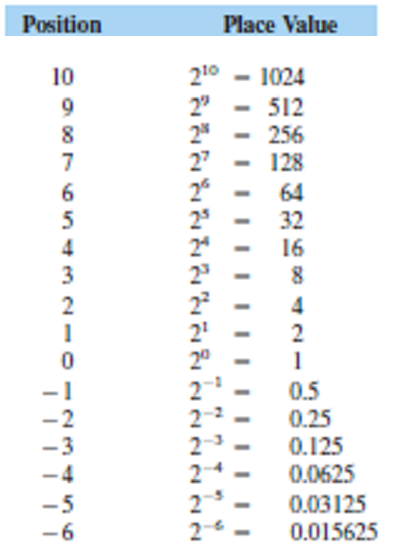Expanded Notation

Thus the value of any digit in a number is the product of that digit and the place value. The value of the entire number is then the sum of these products.

Example 4:

(a) The decimal number 526 can be expressed as

5×102 + 2×101 +6×100

or

5×100+2×10+6×1

or

500+20+6

(b) The binary number 1011 can be expressed as

1×23 +0x22 +1×21 +1×20

or

1×8+0x4+1×2+1×1

or

8+0+2+1

Numbers written in this way are said to be in expanded notation.

### Converting Binary Numbers to Decimal

To convert a binary number to decimal, simply write the binary number in expanded notation (omitting those where the bit is 0), and add the resulting values.

Example 5: Convert the binary number 1001.011 to decimal.

Solution: In expanded notation,

1001.011 = 1×8+1×1+1×1/4 +1×1/8

=8+1+1/4+1/8

=9 3/8

=9.375

Largest Decimal Number Obtainable with n Bits

The largest possible 3-bit binary number is
111=7
or

23 – 1

The largest possible 4-bit number is 1111=15
or

24 – 1

Similarly, the largest n-bit binary number is given by the following:

Largest n-Bit

Binary Number 2n – 1

Example: If a computer stores numbers with 15 bits, what is the largest decimal number that can be represented?
Solution: The largest decimal number is

215 – 1 = 32,767

Significant Digits

In above example we saw that a 15-bit binary number could represent a decimal number no greater than 32,767. Thus it took 15 binary digits to represent 5 decimal digits. As a rule of thumb, it takes about 3 bits for each decimal digit. Stated another way, if we have a computer that stores numbers with 15 bits, we should assume that the decimal numbers that it prints do not contain more than 5 significant digits.

Example: If we want a computer to print decimal numbers containing 7 significant digits, how many bits must it use to store those numbers?

Solution: Using our rule of thumb, we need

3 x 7 = 21 bits

### Converting Decimal Integers to Binary

To convert a decimal integer to binary, we first divide it by 2, obtaining a quotient and a remainder. We write down the remainder and divide the quotient by 2, getting a new quotient and remainder. We then repeat this process until the quotient is zero.

Example: Convert the decimal integer 59 to binary.

Solution: We divide 59 by 2, getting a quotient of 29 and a remainder of 1. Then dividing 29 by 2 gives a quotient of 14 and a remainder of 1. These calculations, and those that follow, can be arranged in a table, as follows: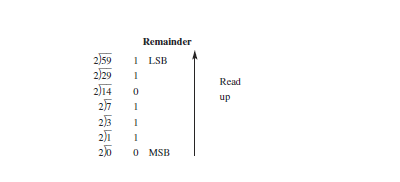Our binary number then consists of the digits in the remainders, with those at the top of the column appearing to the right in the binary number. Thus 5910= 1110112

The conversion can, of course, be checked by converting back to decimal.

To convert a decimal fraction to binary, we first multiply it by 2, remove the integer part of the product, and multiply by 2 again. We then repeat the procedure.

Example: Convert the decimal fraction 0.546875 to binary.

Solution: We multiply the given number by 2, getting 1.09375. We remove the integer part, 1, leaving 0.09375, which we again multiply by 2, getting 0.1875. We repeat the computation until we get a product that has a fractional part of zero, as in the following table: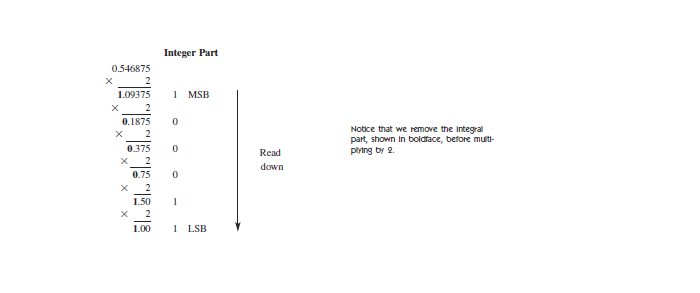We stop now that the fractional part of the product (1.00) is zero. The column containing the integer parts is now our binary number, with the digits at the top appearing at the left of the binary number. So 0.54687510 = 0.1000 112

In this example we were able to find an exact binary equivalent of a decimal fraction. This is not always possible, as shown in the following example.

Example: Convert the decimal fraction 0.743 to binary.

Solution: We follow the same procedure as before and get the following values.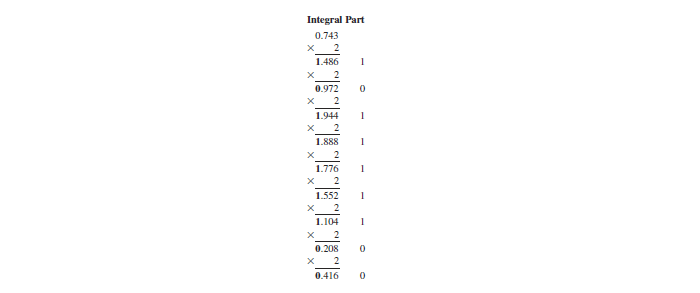It is becoming clear that this computation can continue indefinitely, demonstrating that not all decimal fractions can be exactly converted to binary. This inability to make an exact conversion is an unavoidable source of inaccuracy in some computations.

To decide how far to carry out computation, we use the rule of thumb that each decimal digit requires about 3 binary bits to give the same accuracy. Thus our original 3-digit number requires about 9 bits, which we already have. The result of our conversion is then 0.74310 = 0.1011 1110 02

To convert a decimal number having both an integer part and a fractional part to binary, convert each part separately as shown above, and combine.

Example: Convert the number 59.546875 to binary.

Solution: From the preceding examples,
59  = 0011 1011
and
0.546875 = 0.1000 11

So

59.546875 = 11 1011.1000 11

### Converting Binary Fractions to Decimal

To convert a binary fraction to decimal, we use Table 1 to find the decimal equivalent of each binary bit located to the right of the binary point, and add.

Example: Convert the binary fraction 0.101 to decimal.

Solution: from above table,

0.1     = 2-1 = 0.5
0.001 = 2-3 = 0.125

The procedure is no different when converting a binary number that has both a whole and a fractional part.

Example: Convert the binary number 10.01 to decimal.

Solution: from above table,

10      =  2
0.01   = 0.25

Hexadecimal numbers (or hex for short) are obtained by grouping the bits in a binary number into sets of four and representing each such set by a single number or letter. A hex number one-fourth the length of the binary number is thus obtained.

Base 16

Since a 4-bit group of binary digits can have a value between 0 and 15, we need 16 symbols to represent all of these values. The base of hexadecimal numbers is thus 16. We use the digits from 0 to 9 and the capital letters A to F, as shown in below table.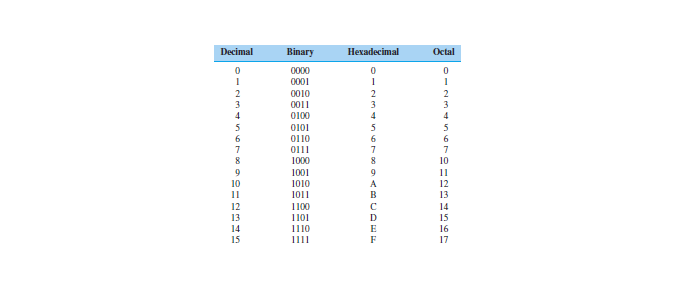To convert binary to hexadecimal, group the bits into sets of four starting at the binary point, adding zeros as needed to fill out the groups. Then assign to each group the appropriate letter or number from above table.

Example 14: Convert 10110100111001 to hexadecimal.
Solution: Grouping, we get
10 1101 0011 1001

or

0010 1101 0011 1001

From above table we obtain
2 D 3 9

So the hexadecimal equivalent is 2D39. Hexadecimal numbers are sometimes written with the subscript 16, as in 2D3916

The procedure is no different for binary fractions.

Solution: Grouping from the binary point gives us

10 1111 . 0011 111

or

0010 1111 . 0011 1110

From Table, we have

2 F . 3 E

or 2F.3E.

To convert hexadecimal to binary, we simply reverse the procedure.

Example: Convert 3B25.E to binary.

Solution: We write the group of 4 bits corresponding to each hexadecimal symbol.

3 B 2 5 . E

0011 1011 0010 0101 . 1110

or

11 1011 0010 0101.111.

As with decimal and binary numbers, each hex digit has a place value, equal to the base, 16, raised to the position number. Thus the place value of the first position to the left of the decimal point is 160 = 1 and the next is 161 = 16 and so on.

To convert from hex to decimal, first replace each letter in the hex number by its decimal equivalent. Write the number in expanded notation, multiplying each hex digit by its place value. Add the resulting numbers.

Example: Convert the hex number 3B.F to decimal.

Solution: We replace the hex B with 11, and the hex F with 15, and write the number in expanded form.

3 B . F
3 11 . 15
(3 x 161) + (11 x 160) + (15 X 16-1)
= 48 + 11 + 0.9375
= 59.9375

To convert decimal to hexadecimal, we repeatedly divide the given decimal number by 16 and convert each remainder to hex. The remainders form our hex number, the last remainder obtained being the most significant digit.

Example: Convert the decimal number 83759 to hex.

Solution:

83759 ÷ 16 = 5234 with remainder of 15

5234 ÷ 16 = 327 with remainder of 2

327 ÷ 16 = 20 with remainder of 7

20 ÷ 16 = 1 with remainder of 4

1 ÷ 16 = 0 with remainder of 1

Changing the number 15 to hex F and reading the remainders from bottom up, we find that our hex equivalent is 1472F.

## The Octal Number System

The octal number system uses eight digits, 0 to 7, and hence has a base of eight. A comparison of the decimal, binary, hex, and octal digits is given in above table.

Binary-Octal Conversions

To convert from binary to octal, write the bits of the binary number in groups of three, starting at the binary point. Then write the octal equivalent for each group.

Example: Convert the binary number 1 1010 0011 0110 to octal.

Solution: Grouping the bits in sets of three from the binary point, we obtain 001 101 000 110 110 and the octal equivalents, 1 5 0 6 6 so the octal equivalent of 1 1010 0011 0110 is 15066.

To convert from octal to binary, simply reverse the procedure shown in above example.

Example: Convert the octal number 7364 to binary.

Solution: We write the group of 3 bits corresponding to each octal digit.

7 3 6 4

111 011 110 100

or 1110 1111 0100.

## BCD Codes

In the beginning of this page we converted decimal numbers into binary. Recall that each bit had a place value equal to 2 raised to the position number. Thus the binary number 10001 is equal to

24 + 20 = 16 + 1 = 17

We shall now refer to numbers such as 10001 as straight binary. With a BCD or binary-coded-decimal code, a decimal number is not converted as a whole to binary, but rather digit by digit. For example, the 1 in the decimal number 17 is 0001 in 4-bit binary, and the 7 is equal to 0111. Thus 17  10001 in straight binary and 17 = 0001 0111 in BCD.

When we converted the digits in the decimal number 17, we used the same 4-bit binary equivalents as in Table 2. The bits have the place values 8, 4, 2, and 1, and BCD numbers written in this manner are said to be in 8421 code. The 8421 code and its decimal equivalents are listed in below table.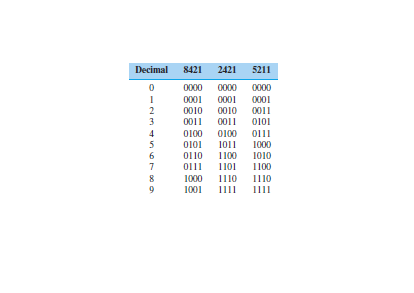Converting Decimal Numbers to BCD

To convert decimal numbers to BCD, simply convert each decimal digit to its equivalent 4-bit code.

Example: Convert the decimal number 25.3 to 8421 BCD code.

Solution: We find the BCD equivalent of each decimal digit from above table.

2  5 . 3

0010 0101 . 0011

or

0010 0101.0011.

Converting from BCD to Decimal

To convert from BCD to decimal, separate the BCD number into 4-bit groups, and write the decimal equivalent of each group.

Example: Convert the 8421 BCD number 1 0011.0101 to decimal.

Solution: We have

0001 0011 . 0101

From above table, we have
1 3 . 5

or

13.5.

Other BCD Codes

Other BCD codes are in use which represent each decimal digit by a 6-bit binary number or an 8-bit binary number, and other 4-bit codes in which the place values are other than 8421. Some of these are shown in table. Each is used in the same way as shown for the 8421 code.

Example: Convert the decimal number 825 to 2421 BCD code.

Solution: We convert each decimal digit separately,

8 2 5

1110 0010 1011

### Other Computer Codes

In addition to the binary, hexadecimal, octal, and BCD codes we have discussed, many other codes are used in the computer industry. Numbers can be represented by the excess-3 code or the Gray code.

There are error-detecting and parity-checking codes. Other codes are used to represent letters of the alphabet and special symbols, as well as numbers. Some of these are Morse code, the American Standard Code for Information Interchange or ASCII (pronounced “as-key”) code, the Extended Binary-Coded-Decimal Interchange Code or EBCDIC (pronounced “eb-si-dik”) code, and the Hollerith code used for punched cards.

Space does not permit discussion of each code. However, if you have understood the manipulation of the codes we have described, you should have no trouble when faced with a new one.

error: Content is protected !!